###### Indexed In
• Genamics JournalSeek
• JournalTOCs
• CiteFactor
• RefSeek
• Hamdard University
• EBSCO A-Z
• OCLC- WorldCat
• Publons

Chicago, USA

### 5th International Conference on Aerospace, Defense and Mechanical Engineering

Amsterdam, Netherlands

### International Conference on Mechanical and Aerospace Engineering

Frankfurt, Germany

### 10th International Conference and Exhibition on Mechanical & Aerospace Engineering

###### Journal Flyer###### Open Access Journals

Research Article - (2015) Volume 4, Issue 6

# Application Framework for Aero-based Design Optimization of Passenger Cars using NURBS

Ghani AO, Agelin-chaab M* and Barari A
Department of Automotive, Mechanical and Manufacturing Engineering, University of Ontario Institute of Technology, Oshawa, Canada
*Corresponding Author: Agelin-chaab M, Department of Automotive, Mechanical and Manufacturing Engineering, Oshawa, Canada, Tel: +1 905-721-8668 Email:

## Abstract

This paper presents a new application framework for aerodynamics-based shape optimization of passenger cars. The rear geometry of a passenger car is the focus of this study due to its significant influence on the aerodynamic characteristics of vehicle. The rear body of a generic car model (the Ahmed body) was represented by Non-Uniform Rational B-Spline (NURBS) curve and NURBS parameters were employed for geometric parameterization. These geometric parameters were systematically modified to alter the geometry using the model developed through a design of experiments process. Computational Fluid Dynamics (CFD) simulations were performed on these geometries to obtain drag coefficients. A polynomial response surface model of drag coefficient was then constructed using linear regression to relate design parameters to the drag coefficient. This response surface model was then used as a starting point for the optimization process. The proposed framework was implemented on a generic notch back car model and the optimized geometric parameters for minimum drag were obtained.

Keywords: CFD; Design optimization; Design of experiments; Response surface modelling; Non-Uniform Rational B-Spline (NURBS)

#### Introduction

In recent years, the improvement of fuel efficiency has become a major factor in passenger car development due to increasing population, global decline in fossil fuel reserves, rising fuel prices and the damaging effects of global warming. The aerodynamic drag of passenger cars is responsible for a large part of a vehicle’s fuel consumption and can contribute to as much as 50% of the total vehicle fuel consumption at highway speeds . Reducing the aerodynamic drag offers an inexpensive solution to improve fuel efficiency and therefore shape optimization for low drag has become an essential part of the overall vehicle design process . Although wind tunnels can provide most realistic data when the test condition are close to actual road condition, the large number of design variables and geometric configurations involved at the conceptual stage of vehicle design make wind tunnel experiments very expensive and time consuming. The availability of high performance computers and relatively accurate turbulence models has led to increased use of CFD in the development of passenger vehicles.

An important aspect of shape optimization through CFD is the parameterization of the model geometry. A common method of parameterization for automotive bodies is the use of geometric parameters such as back light angle (α), boat tail angle (β) and diffuser angle (γ) as shown in Figure 1 [3-5]. Another method is shape modification by displacing particular edges (R1, R2 & R3) on the body in the desired direction as shown in Figure 2 . These parameterization techniques can be implemented in all modern parametric Computer Aided Design (CAD) systems. The drawback of using these parameterization techniques is that only simple shapes with small changes in geometry can be studied. In the present work, the generic notch back model was parameterized using parameters of NURBS curves. The advantage of using NURBS is that it provides a single mathematical formulation to represent a variety of shapes including free form curves and surfaces .

The process usually employed for aerodynamic shape design can be either direct or indirect shape optimization . In the direct shape optimization approach, the process starts with random combinations of design parameters. An optimization algorithm is used which requires CFD simulation at each iteration to find parameters in the design space for minimum drag . This approach requires a large number of CFD simulations and takes significant amount of time to complete the optimization process. On the other hand, in the indirect approach, a design of experiments method is used to obtain geometries from combinations of design parameters and response surface function is built which describes the aerodynamic behaviour of the entire design space. In this study, the indirect shape optimization technique was employed. Linear regression was used to obtain a response surface models that relate the aerodynamic drag coefficient to the NURBS parameters. This response surface model was then used for shape optimization.

#### Background

Mathematical modelling of geometry

The NURBS curves and surfaces have been used extensively in the aerospace industry for parameterizing complex surfaces of wings and fuselages. The NURBS is also the industry standard tool for representing curves and surfaces in CAD, Computer Aided Manufacturing (CAM), and Computer Graphics. Moreover, NURBS is also used for representing curves and surfaces in Initial Graphics Exchange Specification (IGES) which is one of the standard formats to exchange design information between CAD and CAM systems.

Samareh  proposed a free form deformation technique for aerodynamic shape optimization using the NURBS. The optimization was performed on a fuselage of an air plane using the aerodynamic drag coefficient as the objective function. The NURBS parameters changed in their study were the NURBS.

control points. The knot vector and the weights of the control points were kept constant. Lepine  and Bentamy  also performed shape optimization of air foil using NURBS. The design variables in their studies were control points and weights. Lepine  showed that a large number of complex airfoil shapes could be represented using only 13 control points. It was shown that NURBS minimizes the number of design variables and provides smooth profiles. Thus, the main advantage of using NURBS is that free form geometrical shapes can be generated with very few design variables. However, the drawback of using NURBS control points as design variables is the difficulty in changing the relative position of control points, which only allows for the control points to be changed in a small range . In the present study, only the weights of the control points were used for geometric parameterization and it was observed that by careful placement of control points, a large number of geometric variations can be generated. Although NURBS are used in modern CAD software tools for creating free form curves and surfaces, to the best of author’s knowledge, NURBS have never been used for aerodynamics-based automotive body geometric parameterization.

Mathematically, a NURBS curve C(u) of degree p is defined Samareh: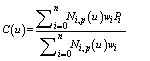(1)

where Ni,p (u) is the B-spline basis function given by: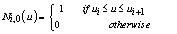(2)(3)

Equation 3 can result in 0/0; which is defined to be zero. The breakpoints of the B-spline are defined by knots and the sequence of knots called a knot vector. There are two fundamental types of knot vectors: clamped and unclamped, which can be either uniform or non-uniform. In uniform knot vector, the individual knots are evenly spaced, whereas non-uniform knot vector may have unequally spaced or multiple internal knots. In clamped knot vector, the knot at the ends has a multiplicity equal to p+1, which is of the form: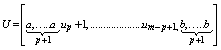(4)

The knot vector U consists of m+1 elements where m is calculated from:(5)

In Equation 4, the first (a) and last (b) elements are repeated p+1 times and are usually set equal to 0 and 1, respectively.

In Equation 1, when the weights of all control points are equal to 1, the resulting curve is a B-spline. The weight of the control point defines how much that control point “attracts” the curve towards itself relative to other control points. Figure 3 shows the effect of changing the weight (h3) of the control point (B3). It can be seen that by modifying the weight of just one control point several different curves can be obtained. This feature of NURBS curves was exploited in this study to generate free form curves that represent the rear geometry of the passenger car. Moreover, NURBS weights were used as design parameters to obtain parametric geometry which was used for shape optimization.

In the present study, a degree 3 NURBS curve with uniform spacing between knots was used. Thus the knot vector for 10 control points using Equations 4 and 5 is:Response surface modeling

Response surface methodology (RSM) is a set of mathematical and statistical techniques used to develop adequate functional relationship between an objective function y(x) and the control or design variables x1, x2, xk . A response surface is a smooth analytical function which is often approximated by lower order polynomials. Mathematically, the approximation can be expressed as:(6)

where y(x) is the unknown function, f(x) is the polynomial function of x, and e is the random error. The two most common models used for RSM are 1st degree and 2nd degree polynomials. The 2nd degree polynomial is used in this study since it has been used successfully for similar problems [13-15]. The model can be expressed as:(7)

where d is the number of design variables and β are the unknown coefficients. In matrix notation, polynomial response surface can be expressed as:(8)

where b is the matrix of unknown coefficients:(9)

Note that β0, βi, βij are the unknown coefficients determined from the least-square regression which minimizes the sum of the squares of the deviations (SSE) of predicted values and the actual values obtained from experiments and xi,xj are the design variables.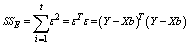(10)

To obtain the polynomial response surface model, a series of experiments need to be performed in which the response variable Y is measured for different combinations of control variables.

#### Design of Experiments

The combinations of control variables are obtained by a systematic procedure called design of experiments. The design of experiments is a concept that uses a set of selected experiments to draw information about the general behavior of the studied object against a set of factors which affect the response . It helps to keep the number of performed experiments as low as possible and to obtain most of information with this set of experiments. For this study, a D-optimal array is used which enables more efficient construction of a quadratic response surface model .

#### Methodology

As stated earlier, the drag characteristics of car strongly depend on the rear geometry. Therefore, only the rear geometry of Ahmed body  was represented with NURBS and parameterized. In addition, the weights of control points of NURBS (from here on referred to as NURBS parameters) curve determine how much a control point attracts the curve. The geometry was parameterized with only the weights of control points. This dramatically reduced the number of design variables and the complexity of parameterization. Since the effect of the control point is local, only the part of the curve in the vicinity of the control point was modified. The accuracy of the fitting model can be assessed by various criteria. The most commonly used criteria are R2 and its adjusted form R2a which also accounts for the number of experiments and degree of freedom.(11)(12)

where t is the number of experiments and r is the number of regression coefficients. SSE and SST are given by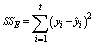(13)(14)

where y is the predicted response. The values of R2 and R2a are between 0 and 1 and the values closer to 1 signify good fit. Another relevant quantity that measures the accuracy of the fit is the Root Mean Squared Error (RMSE).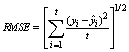(15)

The obtained response surface models must be validated for robustness and accuracy. A common technique is to perform validation experiments over the entire design space and compute the RMSE for validation experiments. The model can then be improved globally by updating the original experiment.

data with the test experiments which have high prediction errors. However, this does not guarantee that the region with optimal design is improved. Another approach is to add the data points with the predicted optimal design to the original experiments and update the model. Unfortunately, it is not obvious which method is preferable since it is very much model and problem dependent [15-18]. The flow chart in Figure 4 outlines the procedure developed by this study for response surface model generation and improvement.

#### Parameterization of Geometry

The geometric parameterization was done using NURBS. The rear body of Ahmed model was represented using 10 control points (P1 to P10). Figure 5 shows the control polygon of the NURBS curve. The number and positions of control points were chosen such that a large variety of shapes could be obtained by changing the NURBS parameters, without the need to change the position of any control point. The weights of the end points W1 and W10 were 1 in all cases since this ensured that the curve passed through the end points. Figure 6 shows a sample notch back geometry which was obtained by setting appropriate values of NURBS parameters also shown in the figure.

#### Numerical Modelling

In order to simplify the problem and reduce computational resources, a two-dimensional computational domain was considered. The rear geometry created using NURBS was imported to ANSYS® Design modeler and attached to the front end of the Ahmed body. The total length (L) and height (H) of the simplified two-dimensional car model were 1.044 m and 0.288 m respectively. To ensure that the domain was sufficiently large for this simulation, the common practical guidelines for automotive external aerodynamics were followed . The domain inlet was 3 model lengths upstream of the model and outlet was 5 model lengths downstream. Thus the total domain was 9 model lengths long. The domain far field was 3 model lengths above the model and the total domain height was 3.3 model lengths.

#### Computational Domain

ANSYS® meshing software in ANSYS® workbench package was used for meshing . Unstructured, non-orthogonal grid with quadrilateral elements was used to create the computational domain. The accuracy of the computational results and the required time directly depend on the number of cells in the computational domain.

The size of the cells near the model should be adjusted such that the wall functions remain valid and the boundary layer should be adequately resolved. Thus a dense mesh is required close to the model and a coarse mesh can be used away from the model. This strategy to divide the computational domain in coarse and fine regions drastically reduced the total cell count and computational time. Figure 7 shows the details of the computational domain.

Figure 7: Numerical domain with boundary layer and grid refinement zones. (a) Boundary layer grid, (b) grid refinement region, and (c) computational mesh.

#### Mathematical Modelling

In this study, the flow was considered to be two-dimensional, incompressible, steady, and turbulent. The fluid was Newtonian with constant density, ρ and dynamic viscosity, μ. The Reynolds Averaged Navier-Stokes (RANS) equations for continuity and momentum conservation can be written as:(16)(17)

whereis the Reynolds stress, pm is the mean flow pressure and ui is the mean flow velocity in the xi direction.

As the goal of this study was to obtain the trends of aerodynamic drag coefficients and to verify the developed framework efficiently, a computationally inexpensive turbulence model was selected. The k-ε model employed in this study is a two equation model based on transport equations for turbulent kinetic energy k and its dissipation rate ε. The k-ε model solves the following two equations of turbulent kinetic energy k and its dissipation rate ε:(18)(19)

where Pk is the production term given by:(20)

and the turbulent viscosity is related to turbulent kinetic energy and dissipation rate by:(21)

The k-ε model constants are

Cε1 =1.44, Cε2 = 1.92, Cμ=0.09, σk=1.0 and σε=1.3 .

#### Boundary Conditions

The inlet velocity must be specified to obtain the desired value of Reynolds number. A velocity of 40 m/s was specified normal to the domain inlet. The Reynolds number based on model length was 2.8 million. In addition, the inlet turbulence intensity was set to 1% and viscosity ratiowas set to 3 since these values are appropriate for external flow analysis . The turbulence intensity is the ratio of the root-mean-square of the velocity fluctuations to the mean flow velocity. When turbulence intensity and viscosity ratio are specified, FLUENT® solver calculates the dissipation rate using the relation:(22)

The outflow condition was specified with zero pressure at the domain outlet. To avoid the effect of shear layers of domain far field on flow field around the model, free-slip wall was specified on domain far field. For car model walls and domain ground, a no-slip boundary condition was imposed.

#### Numerical Simulations

FLUENT® uses finite volume method to discretize the flow equations. The pressure based coupled solver available in FLUENT® was used which solves the momentum and pressure based continuity equations in a coupled manner. This method accelerates the convergence of the solution . For better accuracy of solution, second order discretization was used for discretizing the equations of momentum, kinetic energy and its dissipation rate. The convergence of the solution was based on the drag coefficient as well as the residuals of kinetic energy and dissipation rate. The solution was considered to be converged when there was no change in drag coefficient for at least 100 iterations and the residuals of kinetic energy and dissipation rate were less than 10-6. Moreover, it was also ensured that the dimensionless wall coordinate, y+remained in the desired range (30 < y+ <300) on the car model walls. The average value of y+ for the simulations was approximately 150.

To ensure that the numerical results were independent of the mesh density, mesh independence tests were performed using coarse, medium and fine grids. These were used to estimate the drag coefficient. The change in drag coefficient from the coarse to medium grid was 0.3% and from medium to fine grid was 0.7%. Based on these results a medium grid with approximately 50,000 elements was selected for present study.

#### Shape Optimization of Notch Back

The proposed framework was employed to optimize the aerodynamic shape of a simple notch back model for minimum drag coefficient. The NURBS parameters of top edge of rear window, bottom edge of rear window, boot lid, the base bulge and the diffuser shown in Figure 8 were used for shape optimization and response surface modelling.

To obtain a pure quadratic model with five variables, a total of eleven regression coefficients were needed to be calculated which required at least eleven data points.

Moreover, to acquire enough data for the entire design space, each of the five NURBS parameters was studied at the four levels shown in Table 1. Thus a D-optimal array with 16 runs was used to design the CFD experiments. The drag coefficients obtained from the CFD simulations and the corresponding parameter combinations were then used for linear regression to construct the response surface model.

Parameters Level 1 Level 2 Level 3 Level 4
Rear window top edge W2 0.1 0.5 1 5
Rear window bottom edge W3 0.1 0.5 1 5
Trunk lid W5 0 0.2 0.6 1
Base bulge W7 0.1 0.5 1 5
Diffuser W9 0 0.5 2 10

Table 1: Parameter levels for notch back design.

The parameter levels were chosen considering the changes in the geometry they provided and the sensitivity of the curve. The set of initial 16 CFD simulation experiments performed with different combinations of geometry parameters are shown in Table 1 in the appendix.

A quadratic response model with intercept, linear and quadratic terms of the form of Equation 23 was used to obtain Model 1 for the notch back geometry. Table 2 in the appendix shows the coefficients of Model 1.

Model 1 0.924 0.735 0.0112 0.0098
Model 2 0.834 0.715 0.0107 0.0067

Table 2: Statistics of Model 1 & 2.(23)

The statistics of Model 1 are shown in Table 3 in the appendix. The obtained response surface model was then validated with additional experiments which were designed to test the entire range of parameters and the RMSE was calculated dynamically. A model updating scheme discussed in Figure 4 was applied to reduce the RMSE and the CFD experiments with absolute prediction error greater than 0.01 were added to the fitting data to obtain Model 2 with coefficients shown in Table 4 in the appendix. The validation experiments were performed until the change in RMSE value was considerably small. Table 2 compares the statistics of Models 1 and 2.

 NURBS parameters for minimum Cd Cd W2 W3 W5 W7 W9 0.623 2.875 0.0 0.1 0.0 0.186

Table 3: NURBS parameters for minimum drag coefficient.

It can be seen that Model 2 is superior since it predicts the drag coefficient more accurately over the entire range of design parameters. Figure 9 compares the test RMSE of the two models. It should be noted that although the Model 2 shows better performance globally, it does not guarantee good performance in the region of optimal design.

#### Summary of Results

The constrained non-linear optimization was used to minimize the drag coefficient function represented by Model 2. The starting point of the optimization was Model 2 with all parameters set to level 1 as given in Table 1 with variable bounds between level 1 and level 4. Once the values of parameters were obtained by minimizing the drag coefficient function, CFD simulations were performed with the optimal design parameters and Model 2 was updated with the new data. The process was continued until the drag coefficient value predicted from response surface model and the CFD simulations converged. The developed optimization framework is summarized in the flow chart in Figure 10. The optimization process required 18 iterations to achieve the design parameters for minimum drag coefficient. The optimum design parameters are summarized in Table 3. The optimized rear geometry for minimum drag is shown in Figure 11 and the drag convergence history is shown in Figure 12.

Velocity streamlines and pressure contour around optimized geometry depicted in Figure 13 indicate that the flow separates only at the slanted base and the diffuser geometry at bottom augments the pressure recovery. In case of the high drag geometry obtained during the optimization process, the flow separates at the top edge of the rear window and forms a large recirculation region in the wake as shown in Figure 14. The large recirculation region causes a huge pressure drop in the wake resulting in a higher pressure drag.

Figure 13: Velocity streamlines and pressure contour of minimum drag geometry. (a) Velocity streamlines, and (b) pressure contours.

Figure 14: Velocity streamlines and pressure contour of high drag geometry. (a) Velocity streamlines, and (b) pressure contours.

#### Conclusion

This paper presented a new framework for passenger cars rear geometry parameterization and aerodynamics-based shape optimization. The geometric parameterization was implemented using NURBS parameters. The proposed technique greatly simplifies the parameterization process. It also provides the flexibility to generate free form shapes which cannot be obtained using conventional parameterization techniques employed in automotive body design optimization. The developed methodology uses the proposed parameterization technique to construct response surface models with NURBS parameters as the design variables. It should be noted that the framework was developed using 2D case in order to validate and demonstrate the method. For the results to be practical and applicable, the method should to be applied to 3D cases.

The framework was employed for the aerodynamics-based shape optimization of a simplified notch back model. The response surface method efficiently directs the optimization process since it correctly predicts the parameters of minimum drag as confirmed by the CFD simulations. The proposed framework was implemented successfully using NURBS parameters for a car’s rear geometry aerodynamic shape optimization.

The response surface model of aerodynamic drag was constructed for five design variables and the optimization process required only 18 iterations to obtain the geometric parameters of minimum drag.

#### Acknowledgement

Financial support of this work by the Natural Sciences and Engineering Research Council of Canada is grateful acknowledged.

#### References

1. Mayer W, Wickern G(2011) The New Audi A6/A7 Family - Aerodynamic Development of Different Body Types on One Platform.
2. Han T, Hammond DC, Sagi CJ (1992) Optimization of Bluff Body for Minimum Drag in Ground Proximity .AIAA Journal 30:882-889.
3. Muyl F, Dumas L, Herbert V (2004) Hybrid Method for Aerodynamic Shape Optimization in Automotive Industry. Computers and Fluids 33:849-858.
4. Peddiraju P, PapadopoulousA, Singh R (2009) CAE Framework for Aerodynamic Design Development of Automotive Vehicles. Presented at 3rd ANSA & μETA International Conference.
5. Rogers DF (2001) An Introduction to NURBS with Historical Perspective. Morgan Kaufmann Pub.
6. Samareh JA (2004) Aerodynamic Shape Optimization Based on Free-Form Deformation. AIAA Paper 4630 .
7. Lepine J, Trépanier J, Pepin F (2000) Wing Aerodynamic Optimization Using an Optimized NURBS Geometrical Representation. AIAA paper 0669.
8. Bentamy A, Trépanier JY, Guibault F (2002)Wing Shape Optimization Using a Constrained NURBS Surface Geometrical Representation. Paper presented in ICAS Congress.
9. SongW, Keane AJ (2004) A Study of Shape Parameterization Methods for AirfoilOptimization . Presented In 10th AIAA/ISSMO Multidisciplinary Analysis and Optimization Conference: 2031-2038.
10. Baker CA, Grossman B, Haftka RT, MasonWH,Watson LT (1998) HSCT Configuration Design Space Exploration Using Aerodynamic Response Surface Approximations. AIAA paper 4803 (2004).
11. Krajnovic S (2009) Optimization of Aerodynamic Properties of High-Speed Trains with CFD and Response Surface Models. The Aerodynamics of Heavy Vehicles II: Trucks, Buses, and Trains 197-211.
12. Marjavaara D (2006) CFD Driven Optimization of Hydraulic Turbine Draft Tubes Using Surrogate Models. PhD dissLuleå University of Technology.
13. Alvarez L (2000) Design Optimization Based on Genetic Programming.PhD diss University of Bradford.
14. Sobester A, Leary SJ, Keane AJ (2004) A Parallel Updating Scheme for Approximating and Optimizing High Fidelity Computer Simulations. Structural and Multidisciplinary Optimization27: 371-383.
15. Ahmed SS, Ramm G, Faltin G (1984) Some Salient Features of the Time-Averaged Ground Vehicle Wake. SAE Technical Paper 840300.
16. Lanfrit M (2005) Best Practice Guidelines for Handling Automotive External Aerodynamics with Fluent. Version 1.2. Fluent Deutschland Gmbh.
17. (2010) ANSYS® Academic Research Release 13.0 Help System Workbench User’s Guide ANSYS Inc.
18. (2010) ANSYS® Academic Research Release 13.0 Help System Fluent Theory Guide ANSYS Inc.
Citation: Ghani AO, Agelin-chaab M, Barari A (2015) Application Framework for Aero-based Design Optimization of Passenger Cars using NURBS. J Appl Mech Eng 4:188.

Copyright: © 2015 Ghani AO, et al. This is an open-access article distributed under the terms of the Creative Commons Attribution License, which permits unrestricted use, distribution, and reproduction in any medium, provided the original author and source are credited.